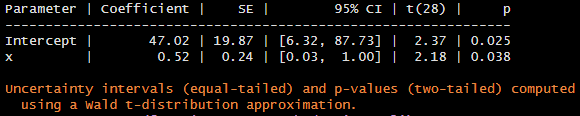# Regression Modeling Strategies: Modeling Longitudinal Responses

This is the seventh of several several connected topics organized around chapters in Regression Modeling Strategies. The purposes of these topics are to introduce key concepts in the chapter and to provide a place for questions, answers, and discussion around the chapter’s topics.

RMS7

1 Like

# Q&A From May 2021 Course

• What do you suggest for panel data? (longitudinal data with time discrete, as often occurs in patients’ registry). No difference. Can use continuous time methods for discrete time, perhaps only changing to an unstructured covariance matrix if there are 2 or 3 distinct times.
• Longitudinal data includes 2 time points? 2 or more
• What if patients were seen at different time-points (clinical routine)?How does it impact time-effect? Continuous-time models such as AR1 handle this nicely.
• How do you decide whether a generalized least square or a mixed effect model fits better your data? Start with the variogram. For a standard mixed-effects models the assumed variogram is flat.
• What is your preferred way to calculate R^2 and/or assess explained variance between fixed and random effects for mixed effect models? Haven’t studied that

Is there a way to run bootstrap validation and calibrate a gls model (fit using gls in the rms package)?

Sorry that’s not implemented.

1 Like

Dear Prof @f2harrell,

I’m trying to simulate some serial data to show the increased Type 1 error rate of analyzing it as if it were independent data.

For instance, I’m comparing the frequency of p<0.05 using OLS vs GLS when there’s no real association between X and Y. The relevant code is as follows:

x <- genCorData(n, mu = c(80, 80, 80),
sigma = 40,
rho = 0.7,
corstr = 'cs',
cnames = c('x1', 'x2', 'x3'))

y <- genCorData(n, mu = c(100, 100, 100),
sigma = 50,
rho = 0.7,
corstr = 'cs',
cnames = c('y1', 'y2', 'y3'))

Gls(y ~ x, data = data, correlation = corCompSymm(form = ~ measure|id)))

Note:

• “cs” above stands for compound symmetry.
• I’m running 1000 simulations of 10 subjects with 3 (x,y) measurements each.

The frequency of p<0.05 is ~ 8% for GLS and ~ 20% for OLS.

Isn’t the first one too high?

I’ll bet that the normal approximation for \hat{\beta} is not great for N=10. The p-value would probably be good were a t-distribution to be used instead of Gaussian.

Thanks.
Do you mean that the fit of the GLS model is suboptimal in terms of achieving normally distributed residuals? Or, alternatively, that the data generating mechanism should be based on sampling X and Y from a t rather than a Gaussian distribution?
Apologies if I’m not following…

Neither. Take the special case of GLS with one time point. Then GLS=OLS and we use the slightly wide t-distribution for getting p-values instead of the normal distribution.

Is there a way to do that using the rms package?

I’ve come across the parameters package:

We can select a t-distribution approximation for computing p-values and confidence limits of regression coefficients:

parameters(f, ci = 0.95, method = 'residual')Is that what you mean?
Unfortunately it does not seem to work for Gls models.

To solve my problem from the message above, I simply increased the sample size from 10 to 30 and now the frequency of p<0.05 is 5.5% with Gls, which seems correct.

The parameters idea is restrictive. A lot of hypotheses are on linear combinations of parameters and involve > 1 degree of freedom. Hence the contrast function in rms. It does’t provide t-based p-values for gls though.

1 Like